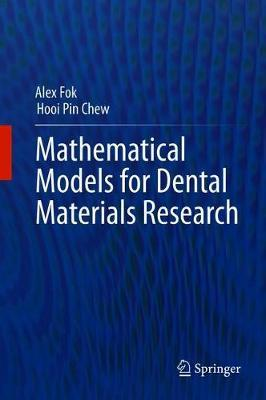# Mathematical Models for Dental Materials ResearchAlex Fok, Hooi Pin Chew

This book presents a mechanistic approach-mathematical modeling-for carrying out dental materials research. This approach allows researchers to go beyond the null hypothesis and obtain a solution that is more general and therefore predictive for conditions other than those considered in a study. Hence it can be used either on its own or to complement the commonly used statistical approach.

Through a series of practical problems with wide-ranging application, the reader will be guided on:

How to construct a mathematical model for the behavior of dental materials by making informed assumptions of the physical, chemical, or mechanical situation
How to simplify the model by making suitable simplifications
How to calibrate the model by calculating the values of key parameters using experimental results
How to refine the model when there are discrepancies between predictions and experiments

Only elementary calculus is required to follow the examples and all the problems can be solved by using MS Excel (c) spreadsheets.

This is an ideal book for dental materials researchers without a strong mathematical background who are interested in applying a more mechanistic approach to their research to give deeper insight into the problem at hand.

Advance praise for Mathematical Models for Dental Materials Research:

“This is a nice addition for research students on how to conduct their work and how to manage data analysis. It brings together a number of important aspects of dental materials investigations which has been missing in the literature. The practical examples make it much easier to understand.” – Michael F. Burrow, Clinical Professor in Prosthodontics, The University of Hong Kong

“The great strengths of this volume are the real world examples of dental materials research in the successive chapters. In turn, this is an outcome of the outstanding expertise of both authors. I warmly recommend this book to the dental biomaterials community worldwide.” – David C. Watts, Professor of Biomaterials Science, University of Manchester, UK